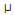# Electronics - Parallel Circuits

Exercise : Parallel Circuits - General Questions
1.
When parallel resistors are of three different values, which has the greatest power loss?
The smallest resistance
The largest resistance
They have the same power loss.
Voltage and resistance values are needed.
Explanation:
No answer description is available. Let's discuss.

2.
What is the product-over-sum result of 150 and 6800?
150
146.7
0.006
6800
Explanation:
No answer description is available. Let's discuss.

3.
The voltage across any branch of a parallel circuit:
varies as the total current varies
is inversely proportional to total circuit resistance
is equally applied to all branch conductances
is dropped in proportion to each branch resistance
Explanation:
No answer description is available. Let's discuss.

4.
What is the total power loss if 2 kand 1 kparallel-connected resistors have an IT of 3 mA?
6W
36W
6 mW
36 mW